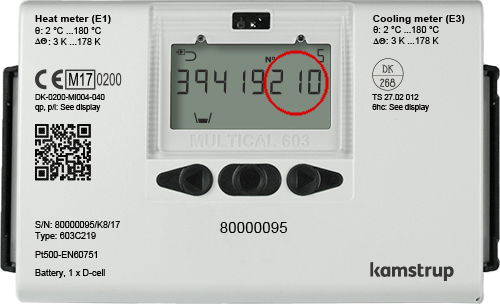#### Interactive User Guides

##### – Heat • Cooling • Water

MULTICAL® 66-CDE

MULTICAL® Compact

MULTICAL® 302

MULTICAL® 401

MULTICAL® 402

MULTICAL® 403

MULTICAL® 601

MULTICAL® 602

MULTICAL® 603

DDD = 110 / 210 / 410

DDD = 110 / 210 / 410 GJ

DDD = 211

DDD = 211 GJ

DDD = 214

DDD = 216

DDD = 216 GJ

DDD = 217

DDD = 217 GJ

DDD = 218

DDD = 218 GJ

DDD = 222

DDD = 222 GJ

DDD = 223

DDD = 223 GJ

DDD = 224

DDD = 224 GJ

DDD = 225

DDD = 225 GJ

DDD = 227

DDD = 227 GJ

DDD = 238

DDD = 238 GJ

DDD = 258

DDD = 258 GJ

DDD = 259

DDD = 259 GJ

DDD = 310 / 610

DDD = 310 / 610 GJ

DDD = 316

DDD = 316 GJ

DDD = 327

DDD = 327 GJ

DDD = 443

DDD = 443 GJ

DDD = 444

DDD = 444 GJ

DDD = 510

DDD = 510 GJ

DDD = 514

DDD = 514 GJ

DDD = 523

DDD = 523 GJ

DDD = 524

DDD = 524 GJ

DDD = 527

DDD = 527 GJ

DDD = 610

DDD = 610 GJ

DDD = 611

DDD = 611 GJ

DDD = 623

DDD = 623 GJ

DDD = 624

DDD = 624 GJ

DDD = 710

DDD = 910

DDD = 910 GJ

DDD = 937

DDD = 937 GJ

DDD = 938

DDD = 938 GJ

DDD = 942

DDD = 942 GJ

DDD = 968

DDD = 995

MULTICAL® 801

MULTICAL® 803

SVM S6

MULTICAL® 61

MULTICAL® 62
– Cold water

MULTICAL® 62
– Hot water

# MULTICAL® 603

## Here you can see how your meter works. Find out with which DDD code your meter has been programmed, and find it on the list in the menu to the left.